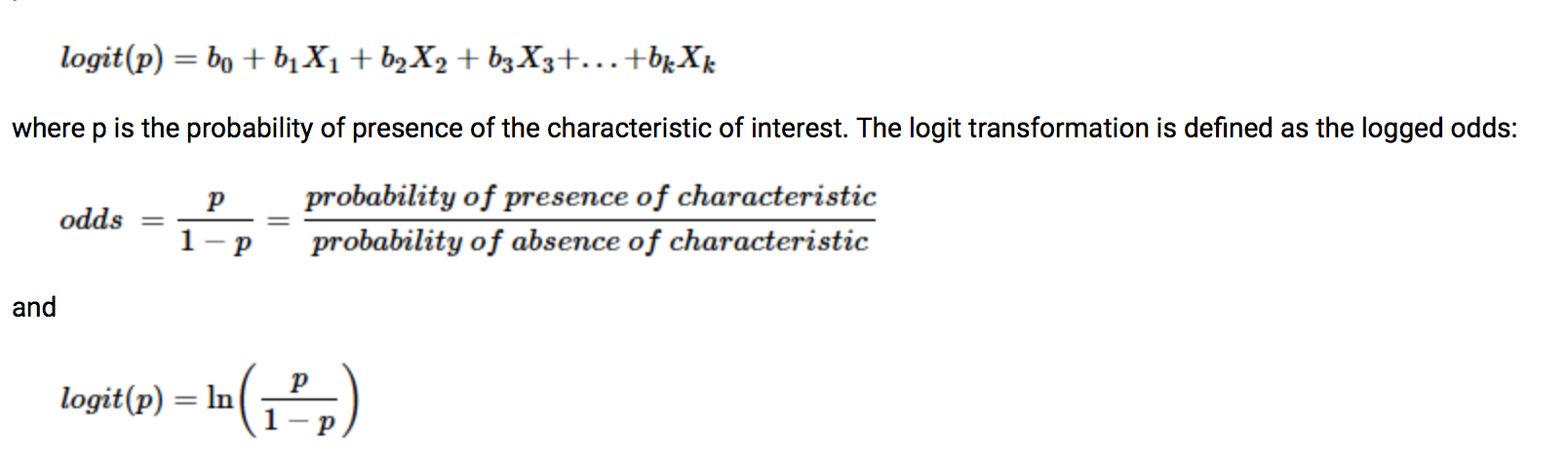19 Jul
19Jul

# Logistic Regression

- In statistics, the logistic model (or logit model) is a statistical model that is usually taken to apply to a binary dependent variable.
- In regression analysis, logistic regression or logit regression is estimating the parameters of a logistic model. Learn More
- Logistic regression is used to describe data and to explain the relationship between one dependent binary or dichotomous variable and one or more nominal, ordinal, interval or ratio-level independent variables.
- In logistic regression, the dependent variable is binary or dichotomous, i.e. it only contains data coded as 1 (TRUE, success, pregnant, etc.) or 0 (FALSE, failure, non-pregnant, etc.).
- Logistic regression generates the coefficients (and its standard errors and significance levels) of a formula to predict a logit transformation of the probability of presence of the characteristic of interest:- Logistic regression uses an equation as the representation, very much like linear regression.
- Input values (x) are combined linearly using weights or coefficient values (referred to as the Greek capital letter Beta) to predict an output value (y). A key difference from linear regression is that the output value being modeled is a binary values (0 or 1) rather than a numeric value.
- Below is an example logistic regression equation:

y = e^(b0 + b1*x) / (1 + e^(b0 + b1*x))
- Where y is the predicted output, b0 is the bias or intercept term and b1 is the coefficient for the single input value (x). Each column in your input data has an associated b coefficient (a constant real value) that must be learned from your training data.

### Comparison to linear regression

- As you may have already realized, linear regression is not the best approach for all problems, specifically those that involve classification. A problem can be deemed a classification problem if the outputs are different discrete outcomes.Learn more

-Given data on time spent studying and exam scores. Linear Regression and logistic regression can predict different things:

• Linear Regression could help us predict the student’s test score on a scale of 0 - 100. Linear regression predictions are continuous (numbers in a range).
• Logistic Regression could help use predict whether the student passed or failed. Logistic regression predictions are discrete (only specific values or categories are allowed). We can also view probability scores underlying the model’s classifications.

### Types of logistic regression

• Binary (Pass/Fail)
• Multi (Cats, Dogs, Sheep)
• Ordinal (Low, Medium, High)

- In statistics, multinomial logistic regression is a classification method that generalizes logistic regression to multiclass problems, i.e. with more than two possible discrete outcomes. That is, it is a model that is used to predict the probabilities of the different possible outcomes of a categorically distributed dependent variable, given a set of independent variables (which may be real-valued, binary-valued, categorical-valued, etc.). Multinomial logistic regression is known by a variety of other names, including polytomous LR, multiclass LR, softmax regression, multinomial logit, the maximum entropy (MaxEnt) classifier, and the conditional maximum entropy model. Learn more

### Logistic regression is applicable, for example, if:

- we want to model the probabilities of a response variable as a function of some explanatory variables, e.g. "success" of admission as a function of gender.
- we want to perform descriptive discriminate analyses such as describing the differences between individuals in separate groups as a function of explanatory variables, e.g. student admitted and rejected as a function of gender
- we want to predict probabilities that individuals fall into two categories of the binary response as a function of some explanatory variables, e.g. what is the probability that a student is admitted given she is a female
- we want to classify individuals into two categories based on explanatory variables, e.g. classify new students into "admitted" or "rejected" group depending on their gender.

### Logistic Regression Assumptions

Logistic regression does not make many of the key assumptions of linear regression and general linear models that are based on ordinary least squares algorithms – particularly regarding linearity, normality, homoscedasticity, and measurement level.

First, logistic regression does not require a linear relationship between the dependent and independent variables.  Second, the error terms (residuals) do not need to be normally distributed.  Third, homoscedasticity is not required.  Finally, the dependent variable in logistic regression is not measured on an interval or ratio scale.

However, some other assumptions still apply.

First, binary logistic regression requires the dependent variable to be binary and ordinal logistic regression requires the dependent variable to be ordinal.

Second, logistic regression requires the observations to be independent of each other.  In other words, the observations should not come from repeated measurements or matched data.

Third, logistic regression requires there to be little or no multicollinearity among the independent variables.  This means that the independent variables should not be too highly correlated with each other.

Fourth, logistic regression assumes linearity of independent variables and log odds.  although this analysis does not require the dependent and independent variables to be related linearly, it requires that the independent variables are linearly related to the log odds.

Finally, logistic regression typically requires a large sample size.  A general guideline is that you need at minimum of 10 cases with the least frequent outcome for each independent variable in your model. For example, if you have 5 independent variables and the expected probability of your least frequent outcome is .10, then you would need a minimum sample size of 500 (10*5 / .10).